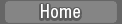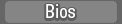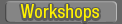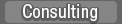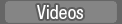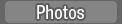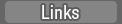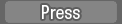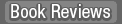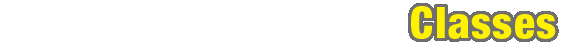The Pridmore System for Card Memorization `My techniques aren't a secret, I'm quite happy to share them with` `anyone who's interested. I normally point people towards the wwbc` `posts last year where I explained it, but since we've got a brand` `new group here, I've rewritten it and made it hopefully a bit easier` `to understand. I don't actually call it the 'Ben system', but that's` `what some people have been calling it, and I do quite like the name.` `It's really just an extension of the basic Major system.` ` ` `The basic principle is the same as everyone else uses, visualising` `images at points along a mental route or journey. I don't use the` `person-action-object ideas of some people, I just have` `three `objects' at each point on my route. Some of these objects are` `people, some are things. I `see' them arranged from left to right,` `or top to bottom, and interacting in various ways according to rules` `I made up as I went along, depending on which objects come together` `in what order.` ` ` `Each object is made from a combination of two playing cards, or` `three decimal digits, or ten binary digits. The name of the object` `starts with a one-syllable sound made up of a consonant, a vowel and` `another consonant. For playing cards, these sounds are made as` `follows.` ` ` `The first consonant is given by the combination of suits, like this:` ` ` `club/club - k` `club/diamond - t` `club/heart - n` `club/spade - m` `diamond/club - r` `diamond/diamond - d` `diamond/heart - l` `diamond/spade - g/j` `heart/club – f/th` `heart/diamond - b` `heart/heart - h` `heart/spade - p` `spade/club - sk/sn/sm` `spade/diamond - st/sp` `spade/heart - sh/sl/sw` `spade/spade – s` ` ` `The vowel comes from the number/rank of the first card, like this:` ` ` `A = `a' as in `cat'` `2 = 'e' as in 'pet'` `3 = 'i' as in 'kitten'` `4 = 'o' as in 'tom'` `5 = 'u' as in 'puss'` `6 = `A' as in `hay'` `7 = `E' as in `bee'` `8 = `I' as in `high'` `9 = `O' as in `low'` `10 = `oo' as in `you'` `J = `ow' as in `cow'` `Q = `or' as in `door'` `K = `ar' as in `car'` ` ` `And the final consonant comes from the number of the second card,` `like this:` ` ` `A = t` `2 = n` `3 = m` `4 = r` `5 = l` `6 = g` `7 = k` `8 = f/th` `9 = b` `10 = s` `J = j/sh/ch` `Q = p` `K = d` ` ` `So, for example, Ace of hearts + 2 of clubs gives f (heart/club) + a` `(Ace) + n (2) = `fan'. If the 2 of clubs came first it would be n` `(club/heart) + e (2) + t (Ace) = `net'.` ` ` `That gives me 2704 different images. For numbers, I use the same` `list of images, but only use 1000 of them for decimal and 1024 for` `binary. It works like this:` ` ` `First consonant (first digit)` ` ` `0 = s` `1 = t` `2 = n` `3 = m` `4 = r` `5 = l` `6 = gj` `7 = k` `8 = f/th` `9 = b` ` ` `Vowel (second digit)` ` ` `0 = `oo'` `1 = `a'` `2 = 'e'` `3 = 'i'` `4 = 'o'` `5 = 'u'` `6 = `A'` `7 = `E'` `8 = `I'` `9 = `O'` ` ` `Second consonant (third digit)` ` ` `0 = s` `1 = t` `2 = n` `3 = m` `4 = r` `5 = l` `6 = g` `7 = k` `8 = f/th` `9 = b` ` ` `And for binary, it's:` ` ` `First consonant (first four digits)` ` ` `0000 = s` `0001 = t` `0010 = n` `0011 = m` `0100 = r` `0101 = l` `0110 = g/j` `0111 = k` `1000 = f` `1001 = b` `1010 = p` `1100 = h` `1101 = sk/sn/sm` `1110 = st/sp` `1111 = sh/sl/sw` ` ` `Vowel (next three digits)` ` ` `000 = `oo'` `001 = `a'` `010 = 'e'` `011 = 'i'` `100 = 'o'` `101 = 'u'` `110 = `A'` `111 = `E'` ` ` `Second consonant (final three digits)` ` ` `000 = s` `001 = t` `010 = n` `011 = m` `100 = r` `101 = l` `110 = g` `111 = k` ` ` `I hope that's clear. I'm always happy to answer questions!`